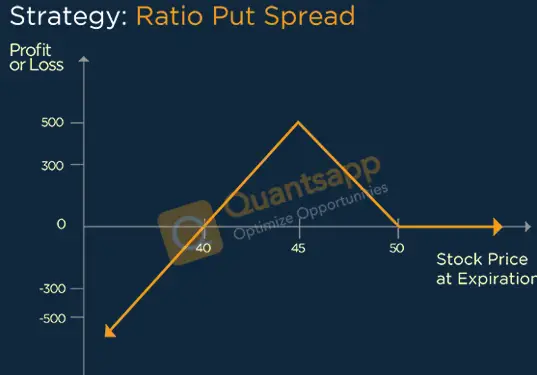# Ratio Put Spread Option Strategy# What is Ratio Put Spread Option Strategy?

• Ratio Put Spread Option Strategy is Neutral to moderately Bearish Strategy. In this we expect stock to remain above lower breakeven point. Ratio Put Spread is a blend of Bear Put Spread and a Naked put sell, where naked Put will be the same as lower strike of Bear Put Spread.

## When to Execute?

• Ratio Put Spread should be implemented when expect slow fall till to the sold strike price and expecting decline in volatility.

• In Ratio Put Spread we generally buy one lot of At-the-money Call and sell two lots of Out-of-money Call option. The variation to Ratio Put spread is to use different ratios such as 1X3,2X3 or 3X5.

• As Net Credit = Lower Strike Price + Difference in strike + Net Premium Received. As net Debit = there will be two breaks even. Upper Breakeven = Lower Strike Price – Difference in strike + Net Premium paid. Lower Breakeven = Higher Strike + Net premium Paid

#### What will be maximum profit?

• Maximum profit would occur at lower strike price at expiry. Here the two short puts will expire worthless and long puts will turn In-the-money (ITM). It can be calculated as follows = Higher Strike – Lower Strike + net premium received.

#### What will be maximum loss?

• Maximum loss is undefined below the lower breakeven because this is not a complete hedge strategy as we short on more put than buying.